150713 Epaper

 A Section B SectionC Section D Section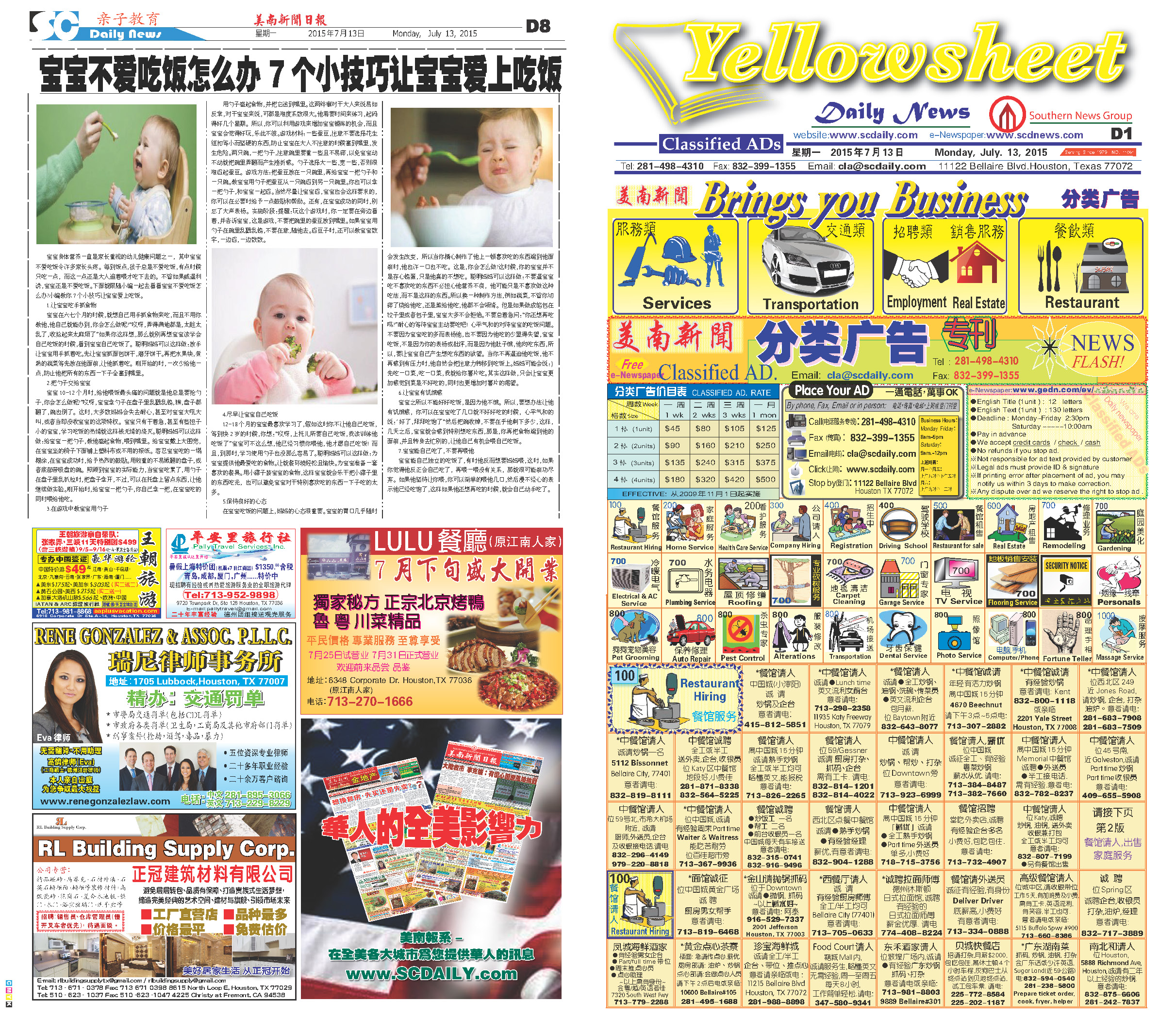150712 Epaper

 A Section B SectionC Section D Section150711 Epaper

 A Section B Section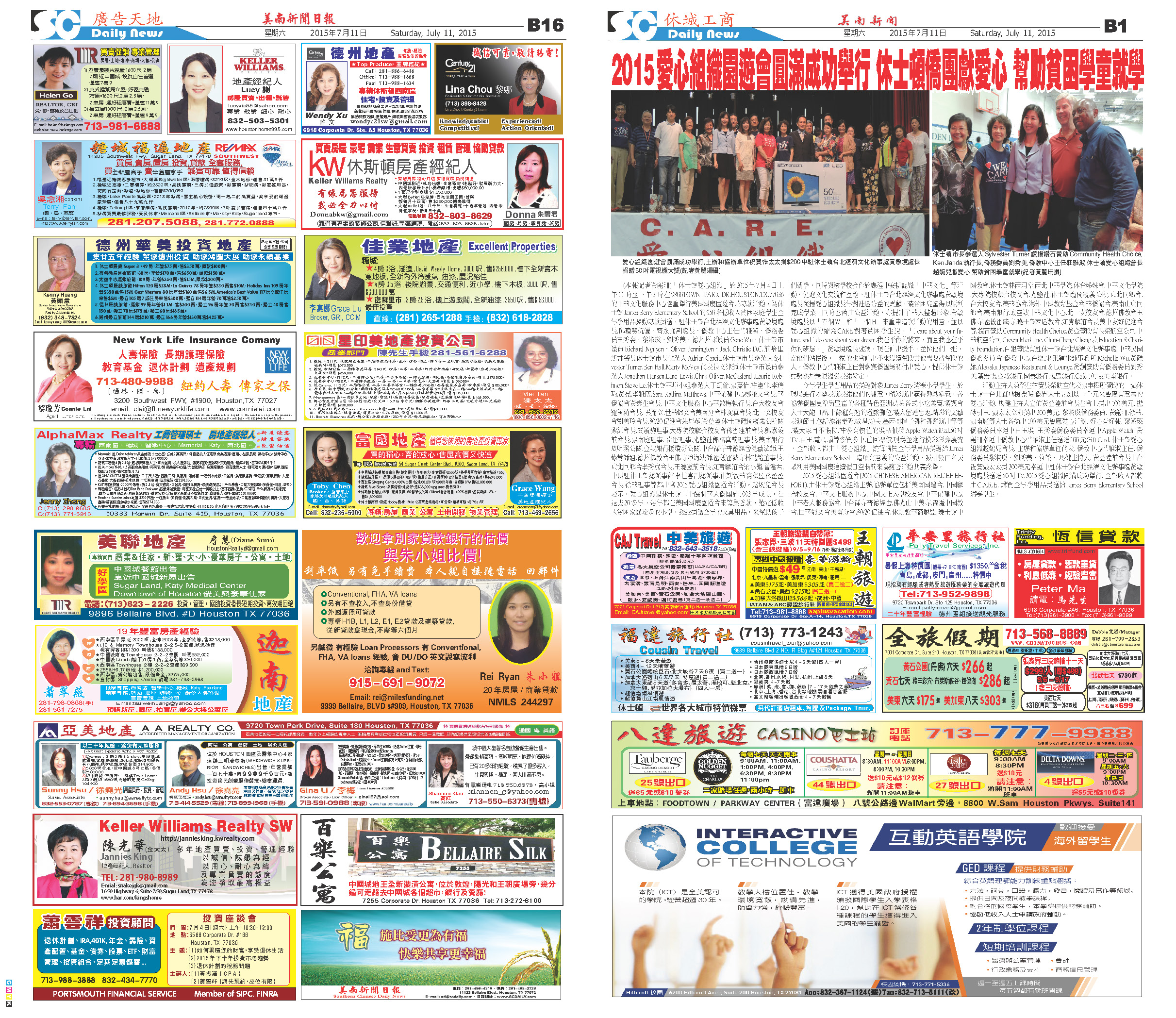C Section D Section150710 Epaper

 A Section B SectionC Section D Section150709 Epaper

 A Section B Section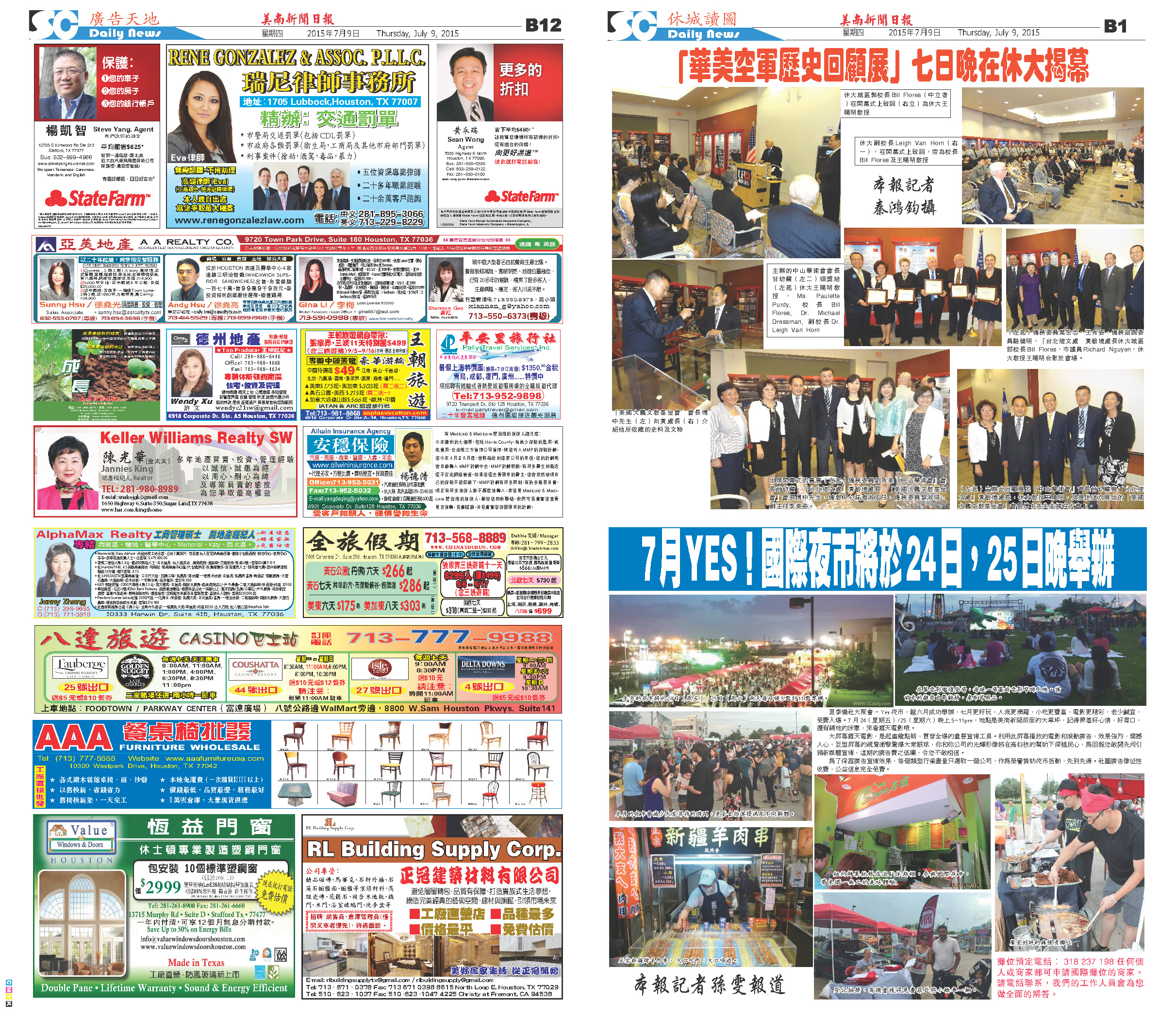C Section D Section150708 Epaper

 A Section B SectionC Section D Section150707 Epaper

 A Section B SectionC Section D Section150706 Epaper

 A Section B SectionC Section D Section150704 Epaper

 A Section B SectionC Section D Section150705 Epaper

 A Section B SectionC Section D Section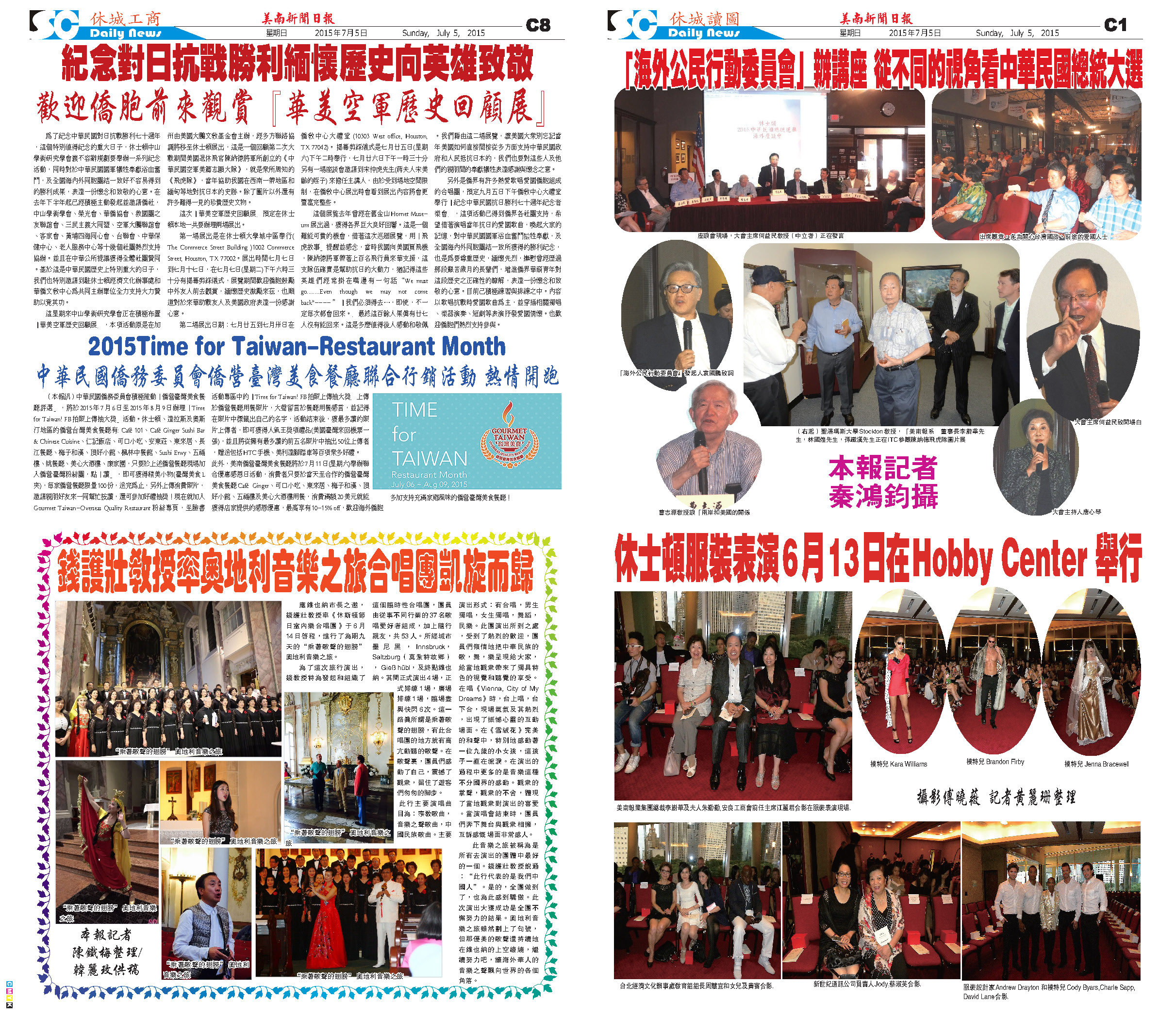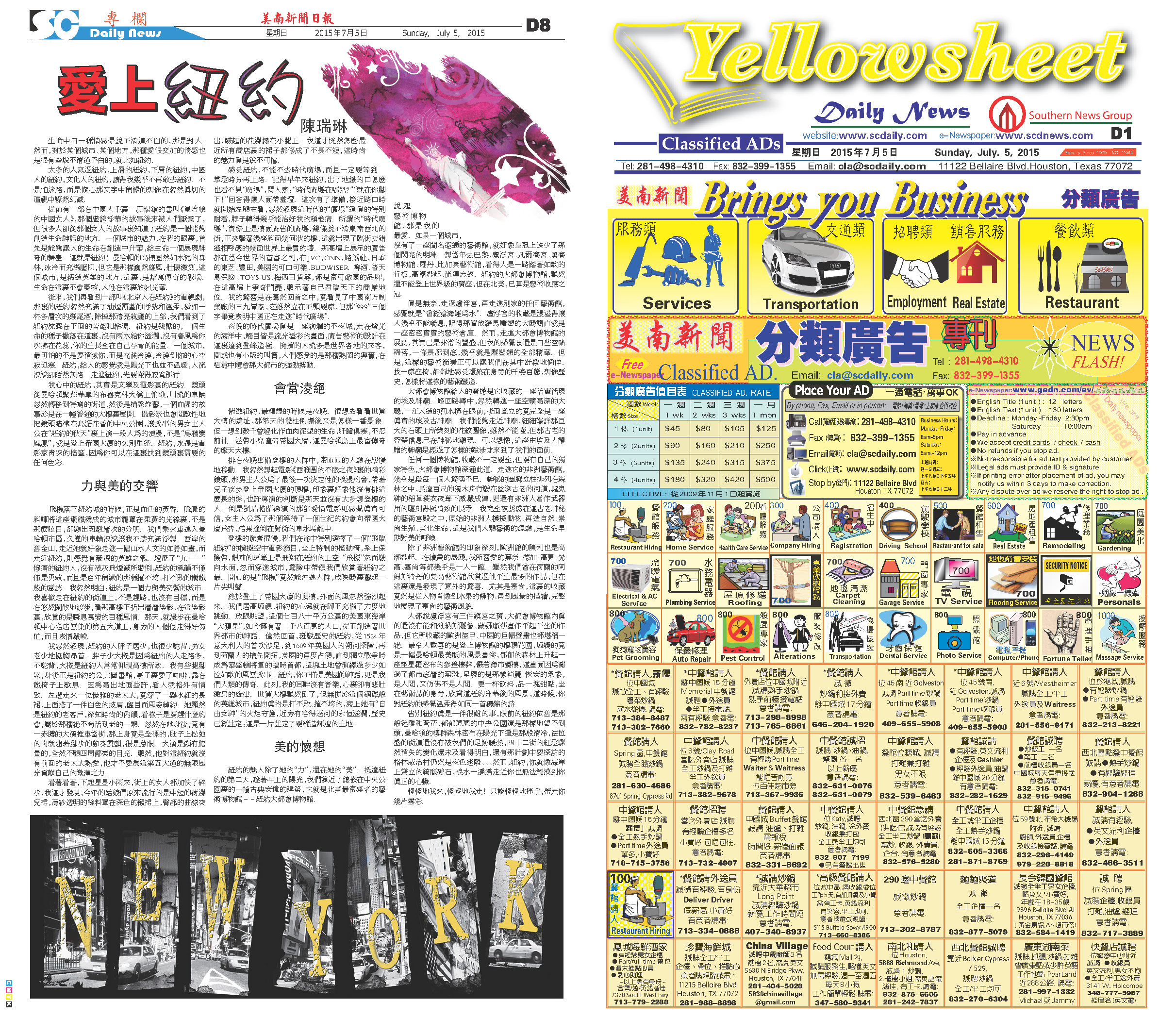150703 Epaper

 A Section B SectionC Section D Section